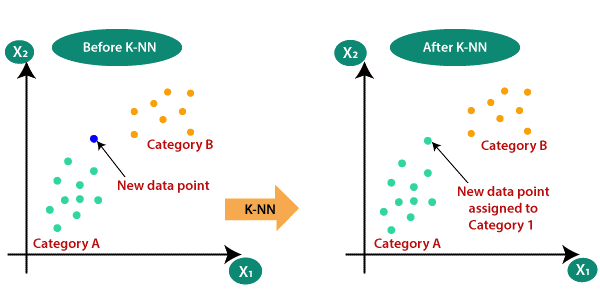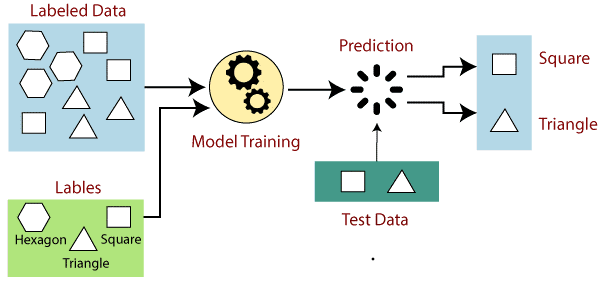## How does supervised machine learning work?

In supervised learning, models are trained using labeled data sets, where the model learns about each type of data. After completing the training process, the model is tested on test-test data (a subset of the training set) and then predicts the output.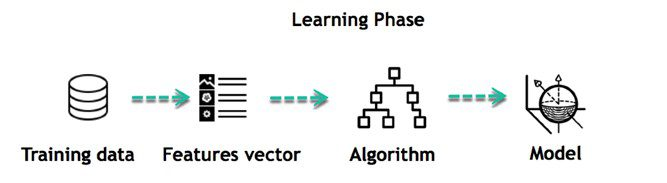## 1. Regression

In regression, a single output value is generated using the training data. This value is a probabilistic interpretation determined after considering the strength of correlation between the input variables. Regression learns from labeled data sets and is then able to predict a continuous-valued output for new data given to the algorithm. For example, regression can predict the price of a house based on its location, size, and etc. help.

Linear Regression – This algorithm assumes a linear relationship between two input variables (X) and output (Y) exists from the data it has learned from. The input variable is called the independent variable and the output variable is called the dependent variable. When unseen data is passed to the algorithm, it uses the function, computes the input, and sums it to a continuous value for the output.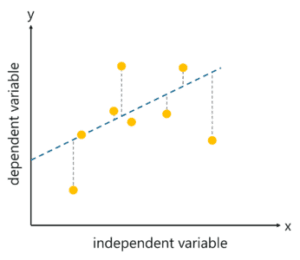Logistic Regression – This algorithm predicts discrete values ​​for a set of independent variables that are passed to it. The algorithm predicts the probability of new data and therefore its output is between 0 and 1.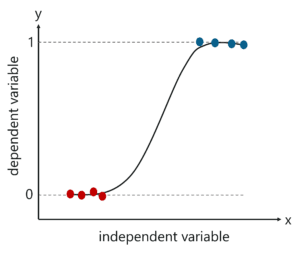## Types of regression: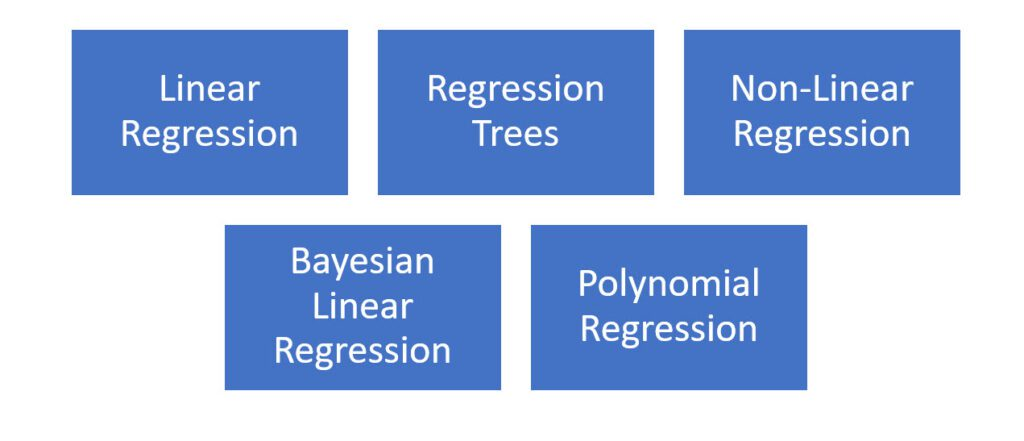## 2. Classification

It involves grouping data into classes. When in classification, the input data is labeled in two separate classes, it is called binary classification. Multiple classification means classifying data into more than two classes. The output will be one of the classes and not the number that was in the regression. Decision trees are classified based on attribute values.
They use the derived information method and figure out which feature of the dataset provides the best information, making it the root node until they can classify each instance of the dataset. Each branch in the decision tree represents an attribute of the dataset. They are one of the most widely used algorithms for classification.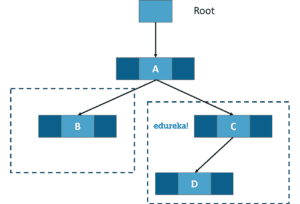## Naive Bayesian Model

Naive Bayes model is used for a large set of data. This is a method of assigning class labels using an acyclic graph. This algorithm assumes that the features of the dataset are all independent of each other. This graph contains a parent node and several child nodes. And each child node is assumed to be independent and separate from the parent.

## Decision Trees

A decision tree is a flowchart-like model that contains conditional control statements that contain decisions and their possible consequences. The output corresponds to the tagging of unpredicted data. In the tree representation, leaf nodes correspond to class labels and internal nodes represent attributes. Decision trees can be used to solve problems with discrete features as well as Boolean functions.

## Support Vector Machines (SVM)

SVM algorithms are based on statistical learning theory. They use Kernal functions, which are a central concept for most learning tasks. These algorithms create a hypermap that is used to classify two classes from each other.## K-Nearest Neighbor

One of the simplest machine learning algorithms is based on the supervised learning technique. The K-NN algorithm assumes the similarity between the new item/data and the existing items and places the new item in the category that is most similar to the existing categories. In the training phase, the KNN algorithm only stores the data set and when it receives new data, it classifies that data into a category that is very similar to the new data.